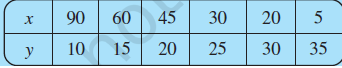Q

# Observe the below table and find which pair of variables (here x and y) are in inverse proportion.

Observe the below table and find which pair of variables (here x and y) are in inverse proportion.

iii)Views

Finding if x and y are in inverse proportion:

Two quantities x and y are said to vary in inverse proportion, if there exists a relation of the type xy = k between them, k being a constant

so, calculating xy for each case,

we have

clearly xy is not equal to a constant value 'k',

hence x and y are not inversely proportional.

Exams
Articles
Questions